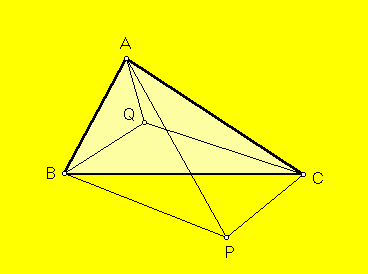# ISOPERIMETRIC POINT AND EQUAL DETOUR POINT

 The isoperimetric point in the plane of a triangle ABC is defined by the trilinear coordinates given just below. For all acute triangles and many others, this point is the unique point P for which the three triangles BPC, CPA, APB have equal perimeters. Trilinear coordinates for the isoperimetric point are sec(A/2) cos(B/2) cos(C/2) - 1 : sec(B/2) cos(C/2) cos(A/2) - 1 : sec(C/2) cos(A/2) cos(B/2) - 1. The equal detour point Q is defined by the trilinears given below. For all acute triangles and many others, Q is the unique point for which the lengths of the 3 detours, A to B via Q, B to C via Q, and C to A via Q, are equal. (The length of the detour A to B via Q means |AQ| + |BQ| - |AB|, and similarly for the other two detours.)Trilinear coordinates for the equal detour point are

sec(A/2) cos(B/2) cos(C/2) + 1 :
sec(B/2) cos(C/2) cos(A/2) + 1 :
sec(C/2) cos(A/2) cos(B/2) + 1.

The isoperimetric point was noted in a problem proposal:

C. Kimberling and R. W. Wagner, Problem E 3020 and Solution, Amer. Math. Monthly 93 (1986) 650-652. [Proposed 1983].

The proposal (1983) led to the introduction of the equal detour point in the following article:

G. R. Veldkamp, "The isoperimetric point and the point(s) of equal detour," Amer. Math. Monthly 92 (1985) 546-558.

(For a quick lesson on trilinears, click on TRILINEAR COORDINATES.)
Triangle Centers